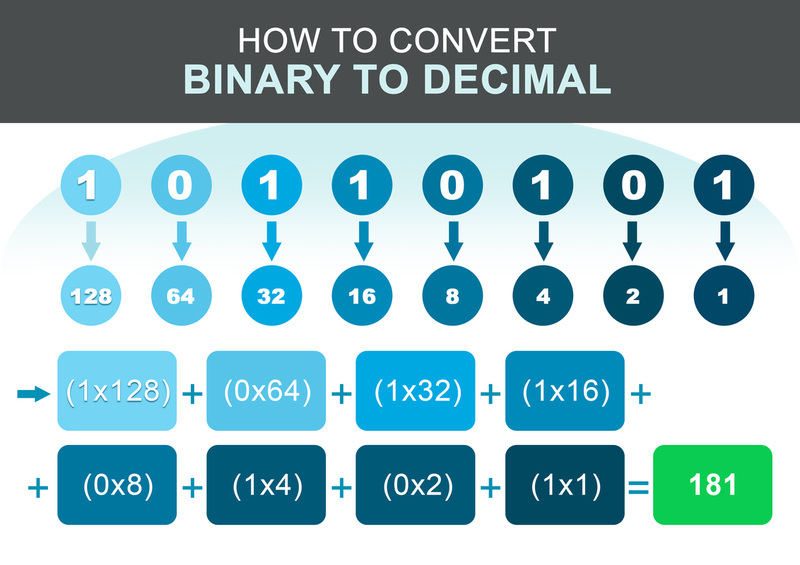Enter Decimal OR Binary Number:
Result:## 如何将二进制数转换为十进制数。

• 第1步：写下二进制数字的每个数字下面的相关重量。权重是数字位置的2次方，从右到左读。
• 第二步：现在注意二进制值等于1的权重。
• 第3步：将上一步中得到的所有数字相加。
• 第四步：上一步的数字将是二进制数字的十进制等值。

1.)第一个步骤。

\begin{array}{|c|c|c|c|c|c|c|} \hline \text{BINARY} & \text{1} & \text{1} & \text{0} & \text{1} & \text{0} & \text{0} & \text{1} \\ \hline \text{相关重量} & \text{64} & \text{32} & \text{16} & \text{8} & \text{4} & \text{2} & \text{1} \\ \hline \end{array}

2.)第二步。 二进制数字为1的权重。

\begin{array}{|c|c|c|c|c|c|c|} \hline \text{64} & \text{32} & \text{8} & \text{1} \\ \hline \end{array}

3.)第三步。将所有的权重相加

$$105 = 64 + 32 + 8 + 1$$

4.)最后一步。二进制的十进制等价物是。: 105

## 如何将十进制数字转换为二进制。

• 第一步：用小数点后的数字除以2，写下余数并赋值R1=余数，同样赋值Q1=本次除法得到的商。
• 第二步：现在用Q1除以2，记下余数。将余数的值分配给R2，将商的值分配给Q1。
• 第3步：继续这个序列，直到在除法的某个时刻，你得到的商（Qn）的值等于0。
• 第4步：你可以把二进制数写成。 $$R(n) R(n-1) . . . . . . . . . . . . . . . . . . . . . R3 R2 R1$$

(179) 小数点 = (10110011) BINARY